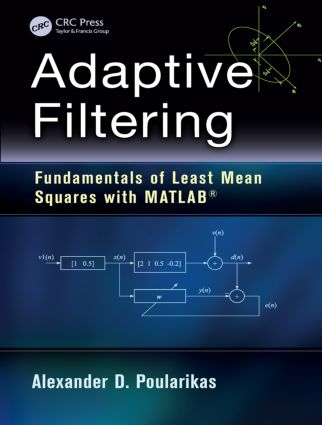## Fundamentals of Least Mean Squares with MATLAB®, 1st Edition

CRC Press

363 pages | 129 B/W Illus.

Paperback: 9781482253351
pub: 2014-09-26
\$115.00
x
Hardback: 9781138417915
pub: 2017-07-27
\$215.00
x
eBook (VitalSource) : 9781315215136
pub: 2017-12-19
from \$57.50

FREE Standard Shipping!

### Description

Adaptive filters are used in many diverse applications, appearing in everything from military instruments to cellphones and home appliances. Adaptive Filtering: Fundamentals of Least Mean Squares with MATLAB® covers the core concepts of this important field, focusing on a vital part of the statistical signal processing area—the least mean square (LMS) adaptive filter.

This largely self-contained text:

• Discusses random variables, stochastic processes, vectors, matrices, determinants, discrete random signals, and probability distributions
• Explains how to find the eigenvalues and eigenvectors of a matrix and the properties of the error surfaces
• Explores the Wiener filter and its practical uses, details the steepest descent method, and develops the Newton’s algorithm
• Addresses the basics of the LMS adaptive filter algorithm, considers LMS adaptive filter variants, and provides numerous examples
• Delivers a concise introduction to MATLAB®, supplying problems, computer experiments, and more than 110 functions and script files

Featuring robust appendices complete with mathematical tables and formulas, Adaptive Filtering: Fundamentals of Least Mean Squares with MATLAB® clearly describes the key principles of adaptive filtering and effectively demonstrates how to apply them to solve real-world problems.

Preface

Author

Abbreviations

MATLAB® Functions

Vectors

Introduction

Multiplication by a Constant and Addition and Subtraction

Unit Coordinate Vectors

Inner Product

Distance between Two Vectors

Mean Value of a Vector

Direction Cosines

The Projection of a Vector

Linear Transformations

Linear Independence, Vector Spaces, and Basis Vectors

Orthogonal Basis Vectors

Problems

Hints–Suggestions–Solutions

Matrices

Introduction

General Types of Matrices

Diagonal, Identity, and Scalar Matrices

Upper and Lower Triangular Matrices

Symmetric and Exchange Matrices

Toeplitz Matrix

Hankel and Hermitian

Matrix Operations

Determinant of a Matrix

Definition and Expansion of a Matrix

Trace of a Matrix

Inverse of a Matrix

Linear Equations

Square Matrices (n × n)

Rectangular Matrices (n < m)

Rectangular Matrices (m < n)

Eigenvalues and Eigenvectors

Eigenvectors

Properties of Eigenvalues and Eigenvectors

Problems

Hints–Suggestions–Solutions

Processing of Discrete Deterministic Signals: Discrete Systems

Discrete-Time Signals

Time-Domain Representation of Basic Continuous and Discrete Signals

Transform-Domain Representation of Discrete Signals

Discrete-Time Fourier Transform

The Discrete FT

Properties of DFT

The z-Transform

Discrete-Time Systems

Linearity and Shift Invariant

Causality

Stability

Transform-Domain Representation

Problems

Hints–Suggestions–Solutions

Discrete-Time Random Processes

Discrete Random Signals, Probability Distributions, and Averages of Random Variables

Stationary and Ergodic Processes

Averages of RV

Stationary Processes

Autocorrelation Matrix

Purely Random Process (White Noise)

Random Walk

Special Random Signals and pdf’s

White Noise

Gaussian Distribution (Normal Distribution)

Exponential Distribution

Lognormal Distribution

Chi-Square Distribution

Wiener–Khinchin Relations

Filtering Random Processes

Special Types of Random Processes

Autoregressive Process

Nonparametric Spectra Estimation

Periodogram

Correlogram

Computation of Periodogram and Correlogram Using FFT

General Remarks on the Periodogram

Proposed Book Modified Method for Better Frequency Resolution

Bartlett Periodogram

The Welch Method

Proposed Modified Welch Methods

Problems

Hints–Solutions–Suggestions

The Wiener Filter

Introduction

The LS Technique

Linear LS

LS Formulation

Statistical Properties of LSEs

The LS Approach

Orthogonality Principle

Corollary

Projection Operator

LS Finite Impulse Response Filter

The Mean-Square Error

The FIR Wiener Filter

The Wiener Solution

Orthogonality Condition

Normalized Performance Equation

Canonical Form of the Error-Performance Surface

Wiener Filtering Examples

Minimum MSE

Optimum Filter (wo)

Linear Prediction

Problems

Hints–Solutions–Suggestions

Eigenvalues of Rx: Properties of the Error Surface

The Eigenvalues of the Correlation Matrix

Karhunen–Loeve Transformation

Geometrical Properties of the Error Surface

Problems

Hints–Solutions–Suggestions

Newton’s and Steepest Descent Methods

Steepest Descent Algorithm

Steepest Descent Algorithm Applied to Wiener Filter

Stability (Convergence) of the Algorithm

Transient Behavior of MSE

Learning Curve

Newton’s Method

Solution of the Vector Difference Equation

Problems

Edition Problems

Hints–Solutions–Suggestions

The Least Mean-Square Algorithm

Introduction

The LMS Algorithm

Examples Using the LMS Algorithm

Performance Analysis of the LMS Algorithm

Learning Curve

The Coefficient-Error or Weighted-Error Correlation Matrix

Stability

The LMS and Steepest Descent Methods

Complex Representation of the LMS Algorithm

Problems

Hints–Solutions–Suggestions

Variants of Least Mean-Square Algorithm

The Normalized Least Mean-Square Algorithm

Power NLMS

Self-Correcting LMS Filter

The Sign-Error LMS Algorithm

The NLMS Sign-Error Algorithm

The Sign-Regressor LMS Algorithm

Self-Correcting Sign-Regressor LMS Algorithm

The Normalized Sign-Regressor LMS Algorithm

The Sign–Sign LMS Algorithm

The Normalized Sign–Sign LMS Algorithm

Variable Step-Size LMS

The Leaky LMS Algorithm

The Linearly Constrained LMS Algorithm

The Least Mean Fourth Algorithm

The Least Mean Mixed Norm LMS Algorithm

Short-Length Signal of the LMS Algorithm

The Transform Domain LMS Algorithm

Convergence

The Error Normalized Step-Size LMS Algorithm

The Robust Variable Step-Size LMS Algorithm

The Modified LMS Algorithm

Momentum LMS

The Block LMS Algorithm

The Complex LMS Algorithm

The Affine LMS Algorithm

The Complex Affine LMS Algorithm

Problems

Hints–Solutions–Suggestions

Appendix 1: Suggestions and Explanations for MATLAB Use

Suggestions and Explanations for MATLAB Use

Creating a Directory

Help

MATLAB as Calculator

Variable Names

Complex Numbers

Array Indexing

Extracting and Inserting Numbers in Arrays

Vectorization

Windowing

Matrices

Producing a Periodic Function

Script Files

Functions

Complex Expressions

Axes

2D Graphics

3D Plots

General Purpose Commands

Managing Commands and Functions

Managing Variables and Workplace

Operators and Special Characters

Control Flow

Elementary Matrices and Matrix Manipulation

Elementary Matrices and Arrays

Matrix Manipulation

Elementary Mathematical Functions

Elementary Functions

Numerical Linear Algebra

Matrix Analysis

Data Analysis

Basic Operations

Filtering and Convolution

Fourier Transforms

2D Plotting

2D Plots

Appendix 2: Matrix Analysis

Definitions

Special Matrices

Matrix Operation and Formulas

Eigendecomposition of Matrices

Matrix Expectations

Differentiation of a Scalar Function with respect to a Vector

Appendix 3: Mathematical Formulas

Trigonometric Identities

Orthogonality

Summation of Trigonometric Forms

Summation Formulas

Finite Summation Formulas

Infinite Summation Formulas

Series Expansions

Logarithms

Some Definite Integrals

Appendix 4: Lagrange Multiplier Method

Bibliography

Index